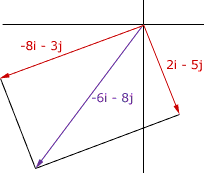Quandaries and Queries Question: I have never been good a word problems. Please help. Two teams are playing push ball with a large 8 foot diameter ball. One team exerts a force represented by the vector a = 2i + -5j, and the other team exerts a force represented by the vector b = -8i-3j. 1.Determine the direction of movement of the ball if the i axis is due east. 2.Determine the combined force magnitude. Hi Brian, I drew a vector diagram showing 2i - 5j and -8i - 3j. The force acting on the ball is the sum of these two vectors (2i - 5j) + (-8i - 3j) = -6i - 8jThe magnitude of the combined force is sqrt[(-6)2 + (-8)2] =sqrt[36 +64] = sqrt = 10 I don't know what units to give this as you didn't give me units for the force vectors. Harley Go to Math Central